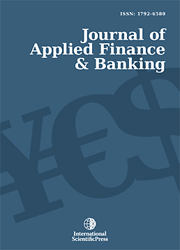# Journal of Applied Finance & Banking

#### Did Linear Stochastic Processes predict accurately Short Term Interest Rate Intertemporal Behaviour?

•[ Download ]
• Times downloaded: 2655
• Abstract

This article attempts to identify the best model of the short term interest rates that can predict its stochastic process over time. We studied nine different models of the short term interest rates. The choice of these models was the aim of analyzing the relevance of certain specifications of the short term interest rate stochastic process, the effect of mean reversion and the sensitivity of the volatility to the level of interest rate. The yield on US three months treasury bills is used as a proxy for the short term interest rates. The parameters of the different stochastic process are estimated using the generalized method of moments. The results show that the effect of mean reversion is not statistically significant and that volatility is highly sensitive to the level of interest rates. To further study the performance prediction of the intertemporal behavior of the short term interest rate of the various models; we simulated their stochastic process for different periods. The results show that none of the studied models reproduce the actual path of the short term interest rates. The problem lies in the parametric specification of the mean and volatility of the diffusion process. To further study the accurate parametric specification of the interest rate stochastic process we use a nonparametric estimation of the drift and the diffusion functions. The results prove that both should be nonlinear.ISSN: 1792-6599 (Online)
1792-6580 (Print)